# Lakhmir Singh Solutions of Class 9 Chemistry Chapter 4 Structure of Atom

Atoms and molecules are building blocks of matter. All mater is made up of atoms and molecules. Atoms have smaller particles in them which are called subatomic particles- electrons, protons, and neutrons. Electrons are negatively charged species, protons are positively charged species and neutrons are neutral which are chargeless. Protons and neutrons are present in a small nucleus at the centre of the atom and electrons are outside the nucleus. The atoms of different elements differ in the number of electrons, protons, and neutrons.

The existence of protons in the atom is shown by E. Goldstein. He found that protons are positively charged particles found in the atom of all elements. James Chadwick discovered the neutral species called neutrons. J.J. Thomson proposed the model of an atom which is similar to a pudding model. There were drawbacks to this model. Rutherford discovered the concept of the nucleus and this too had many drawbacks. Later Neil Bohr explained an acceptable model of the atom.

This chapter covers all the atomic models proposed by different scientists in detail and the terms related to the atom are also explained clearly.

## Download PDF Of Lakhmir Singh Solutions of Class 9 Chemistry Chapter 4 Structure of Atom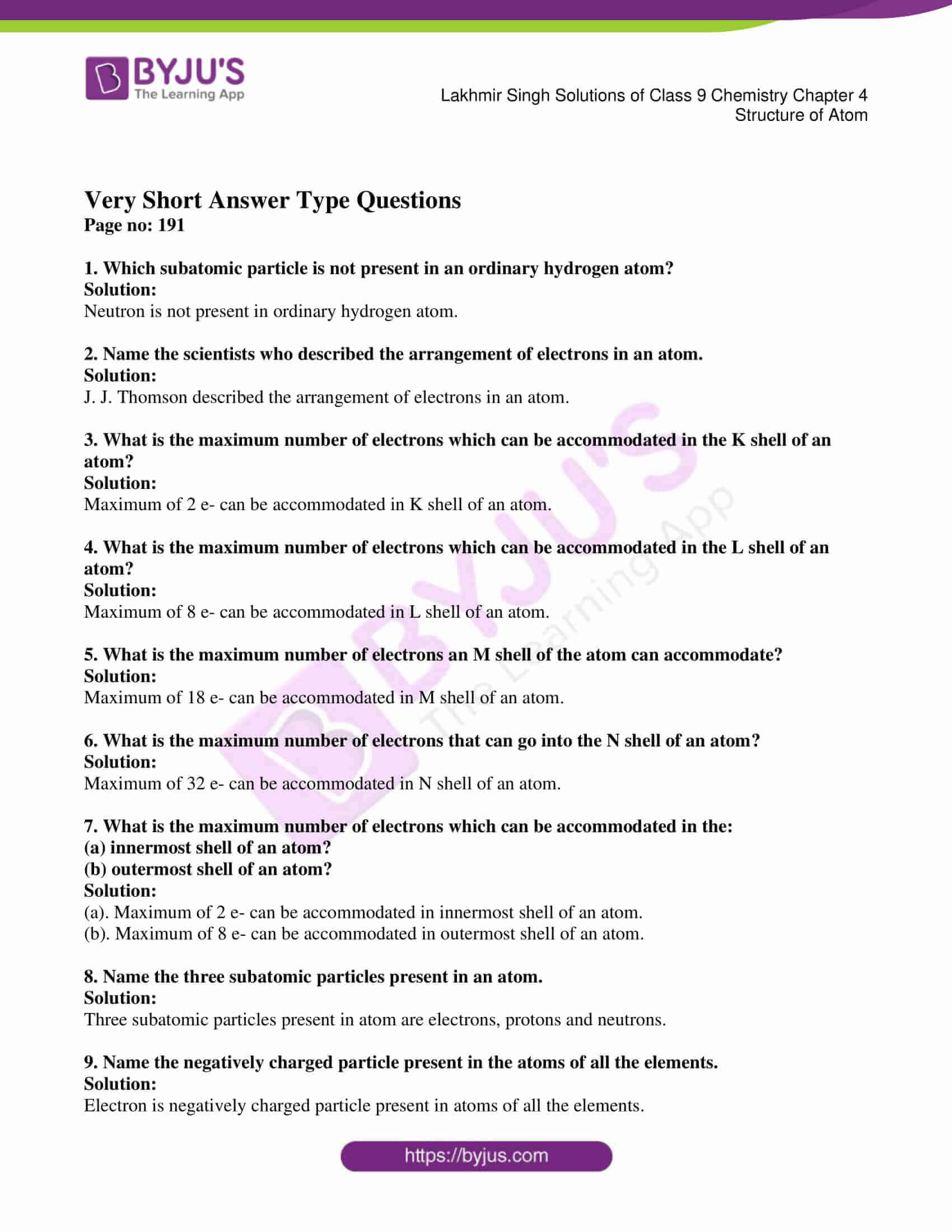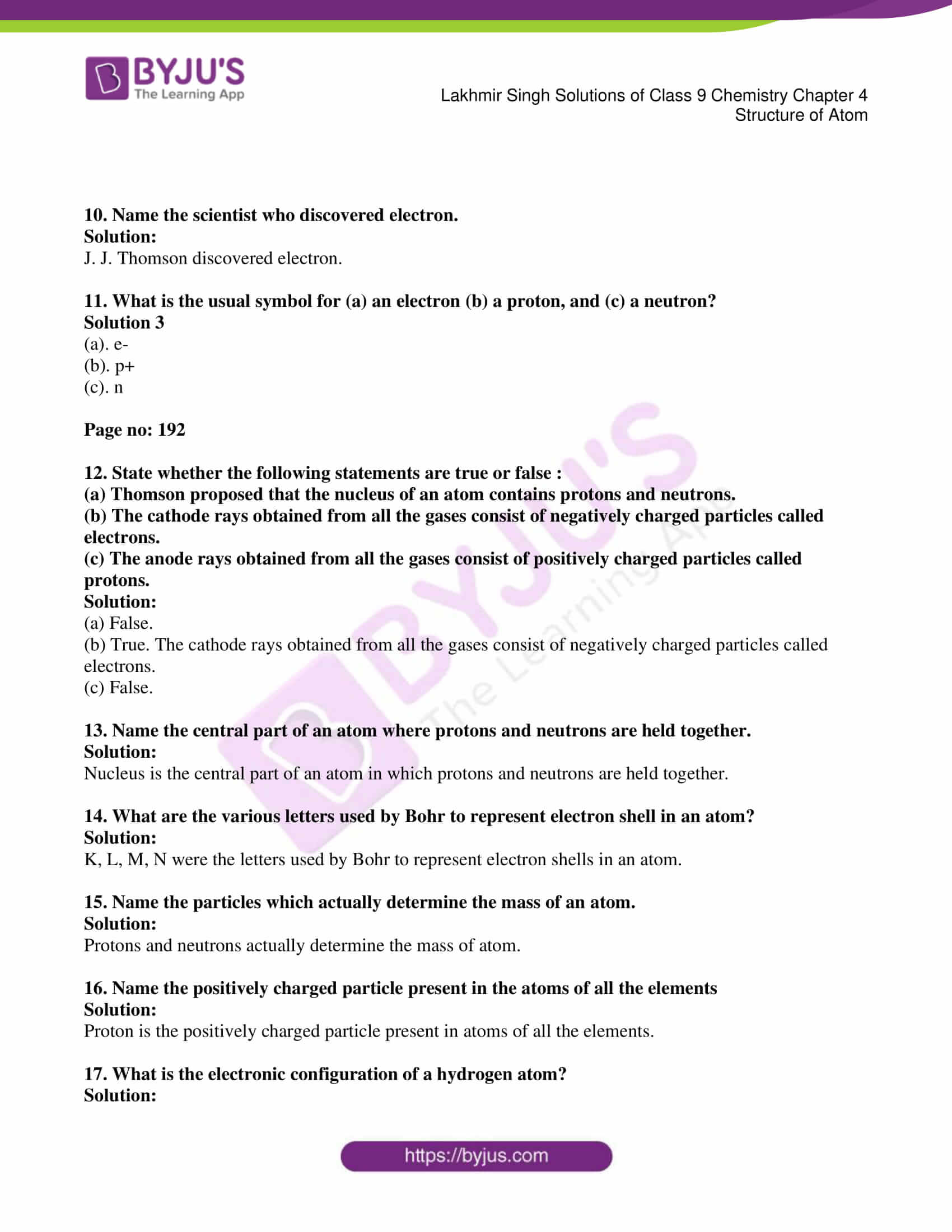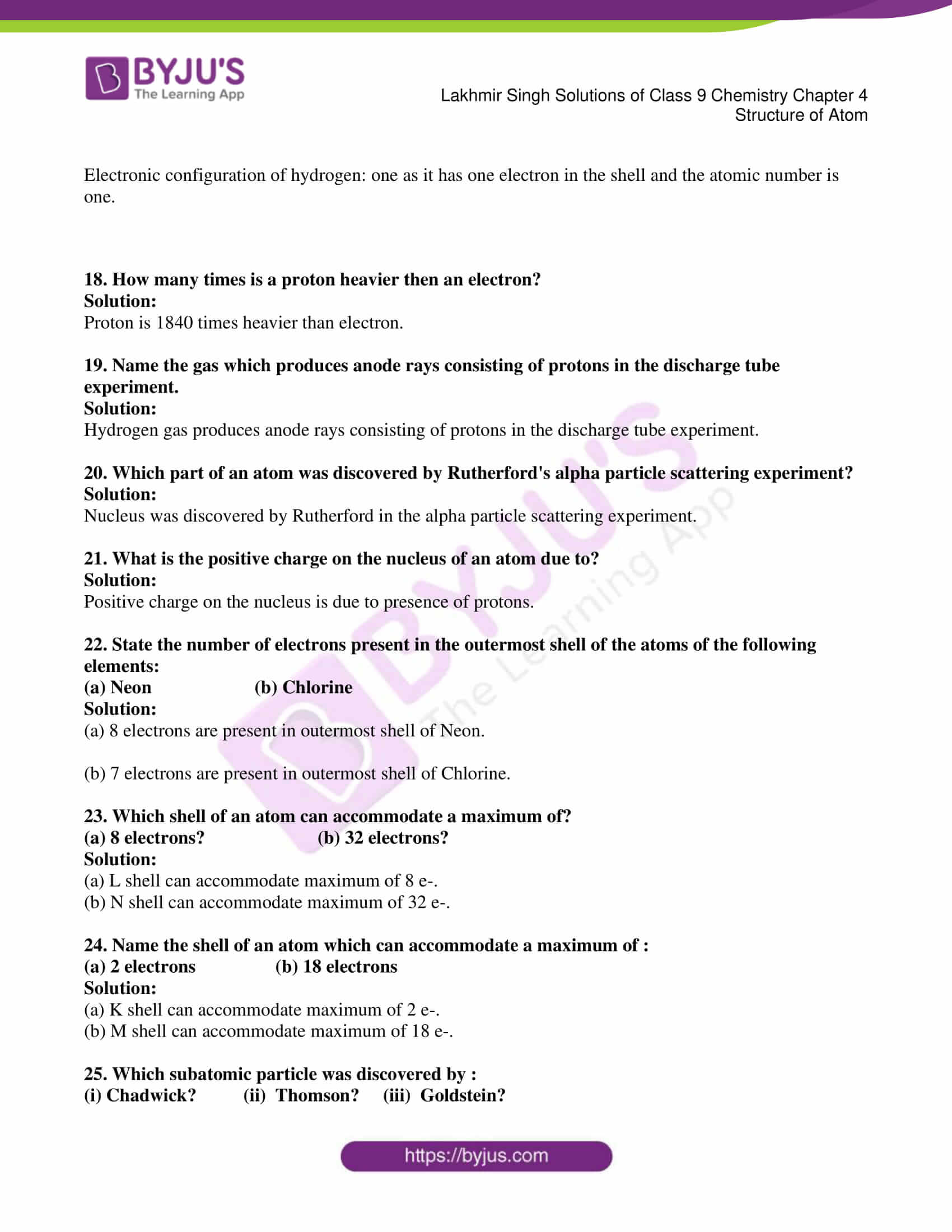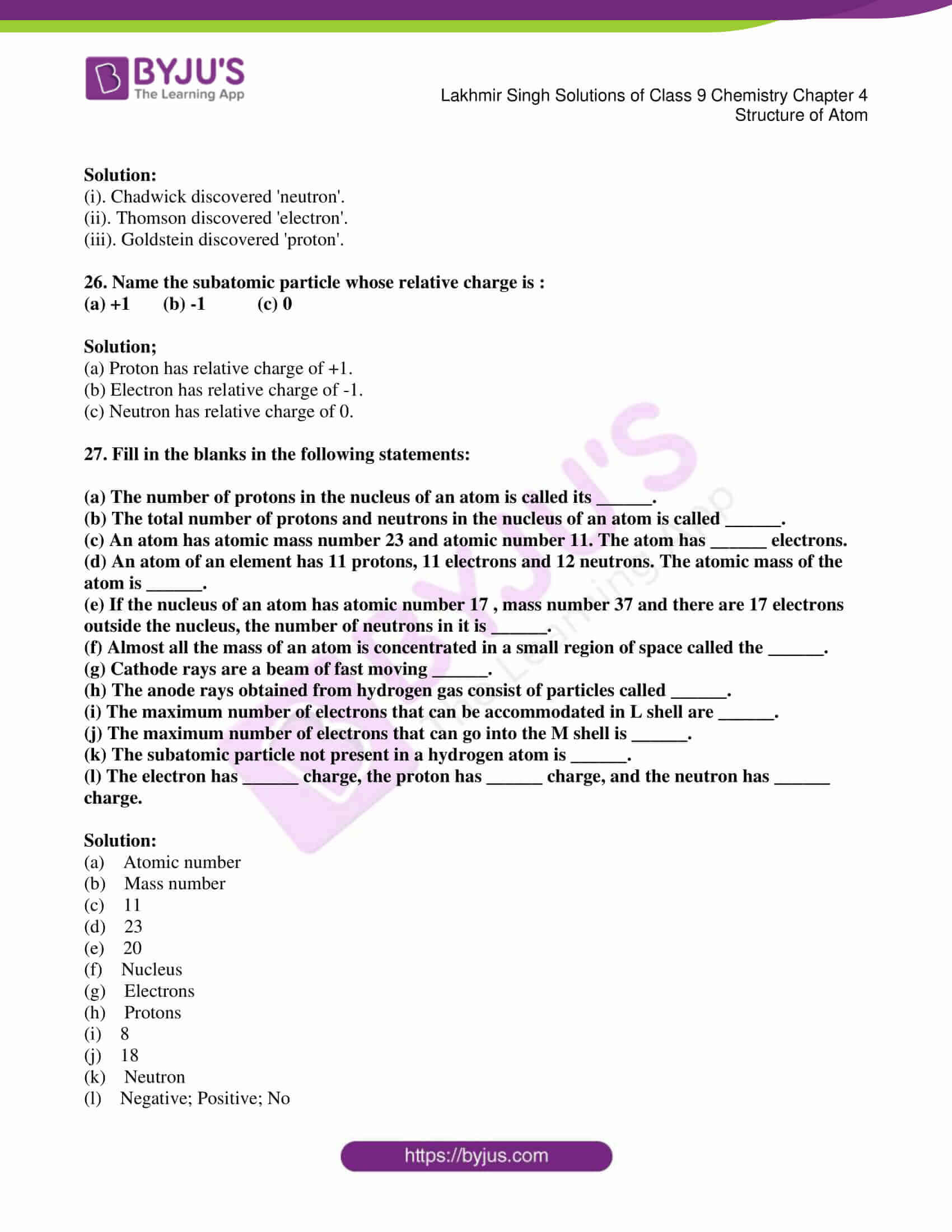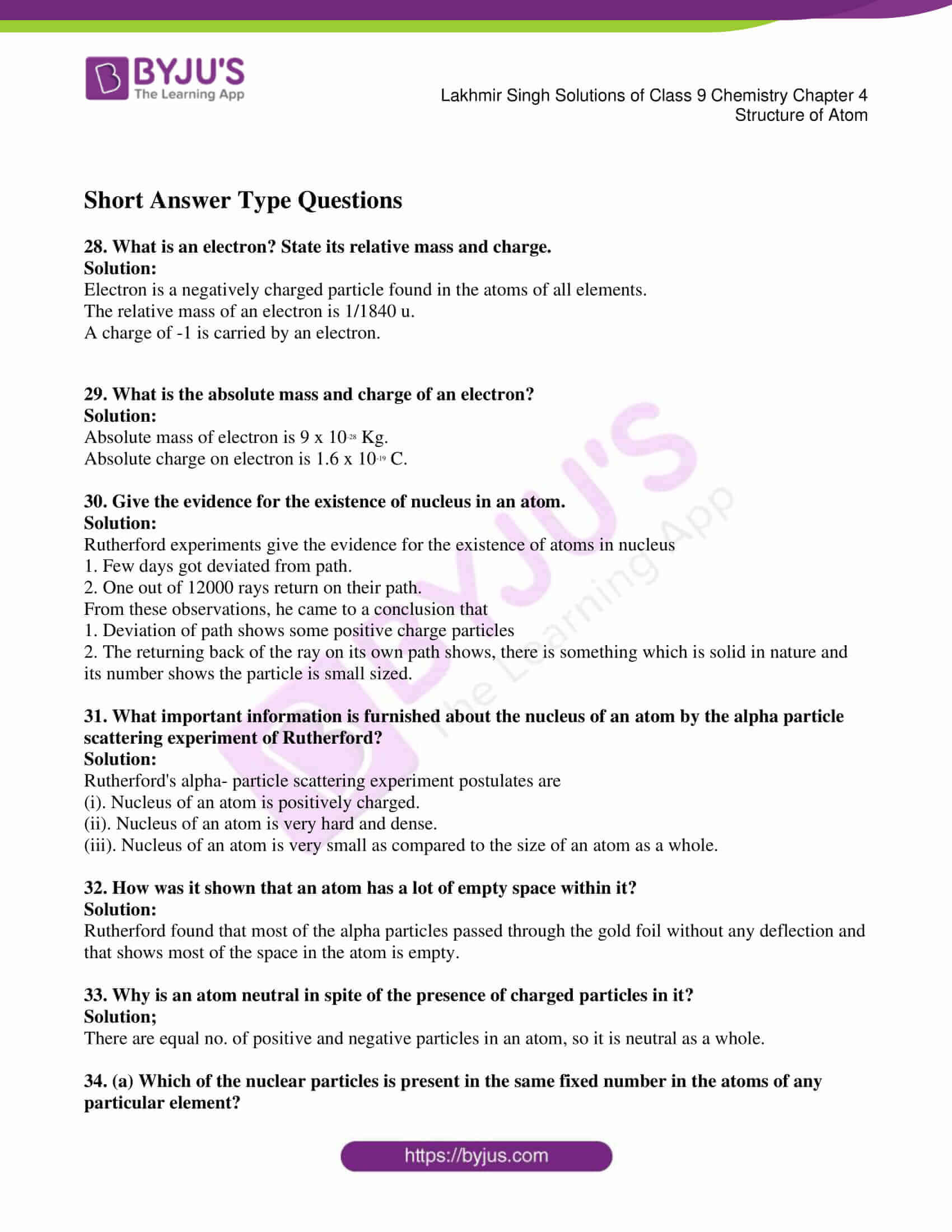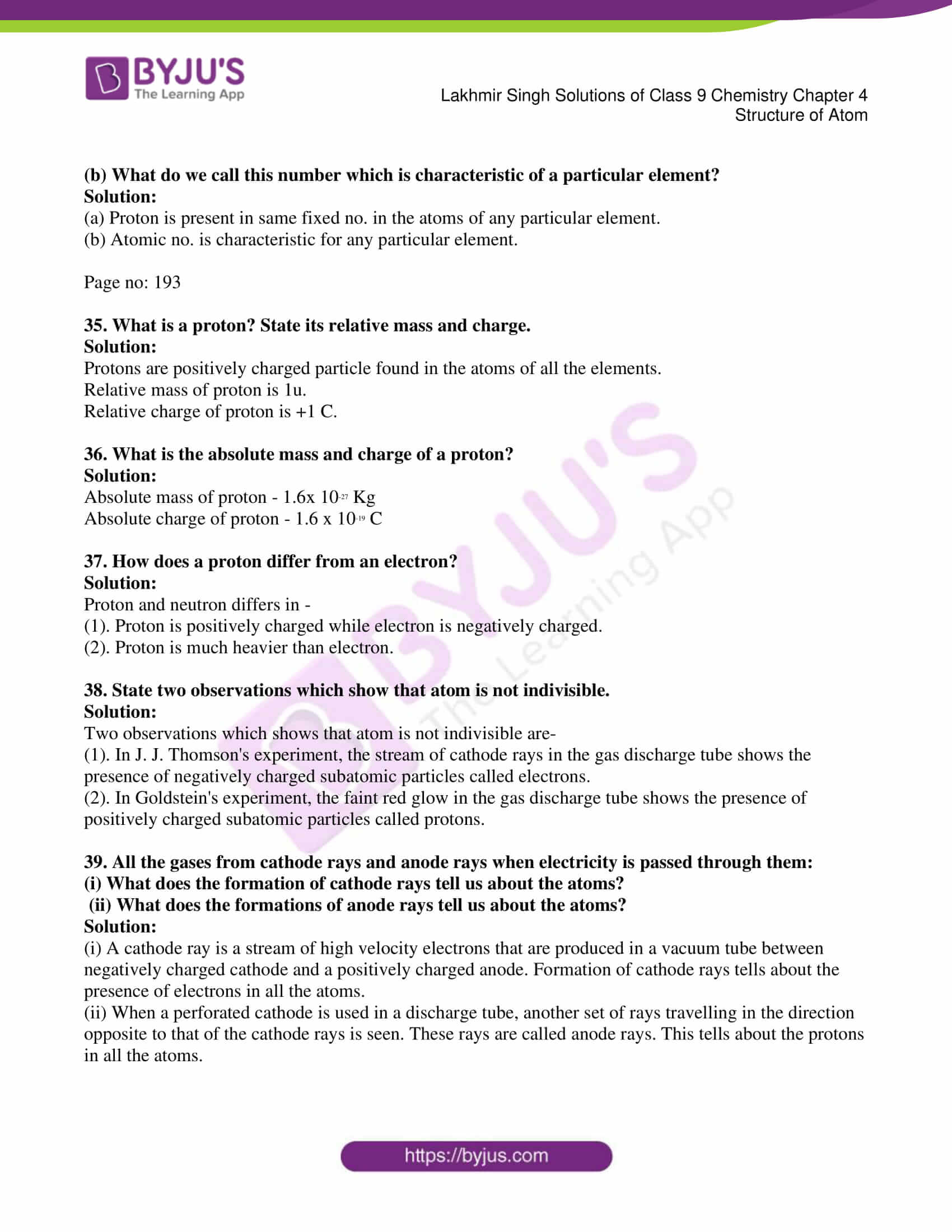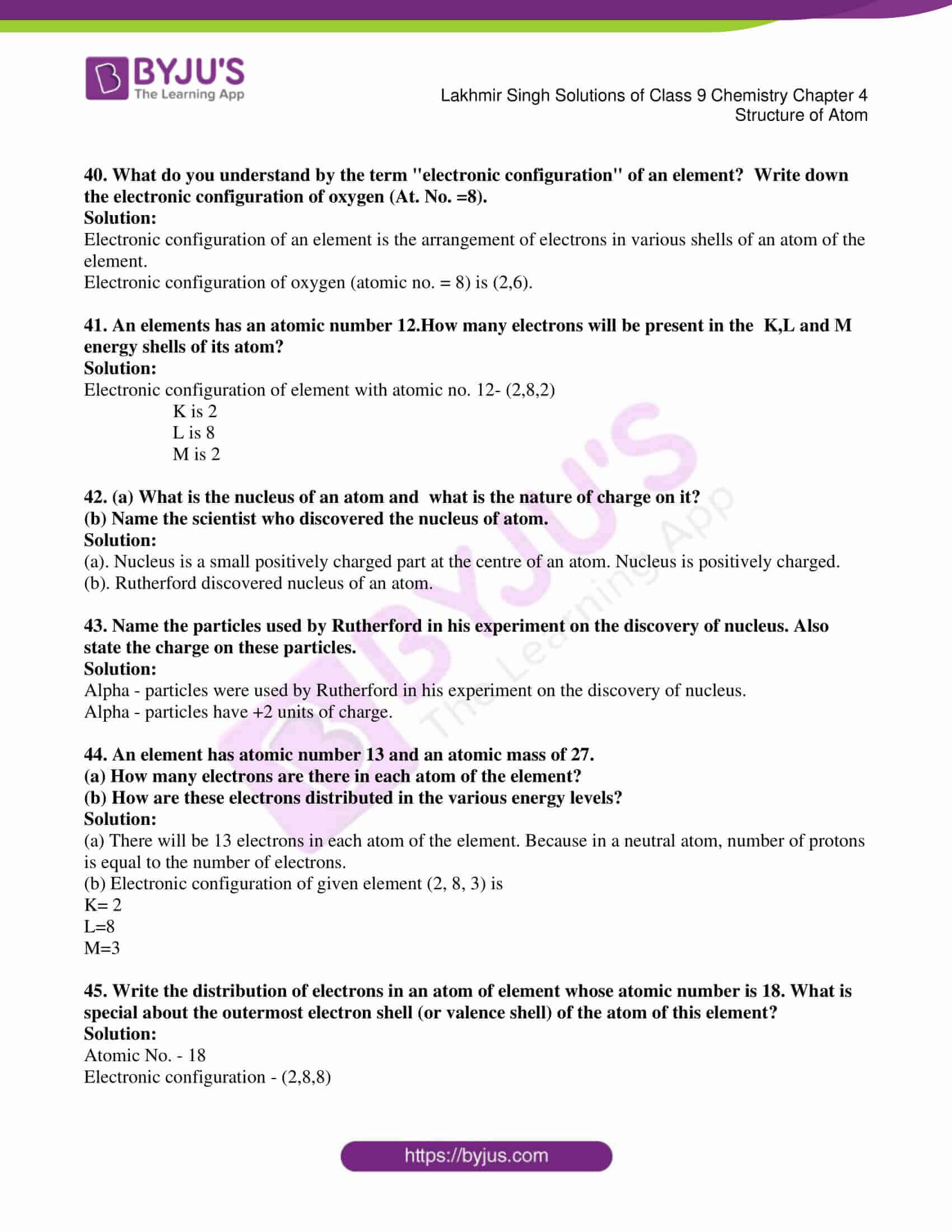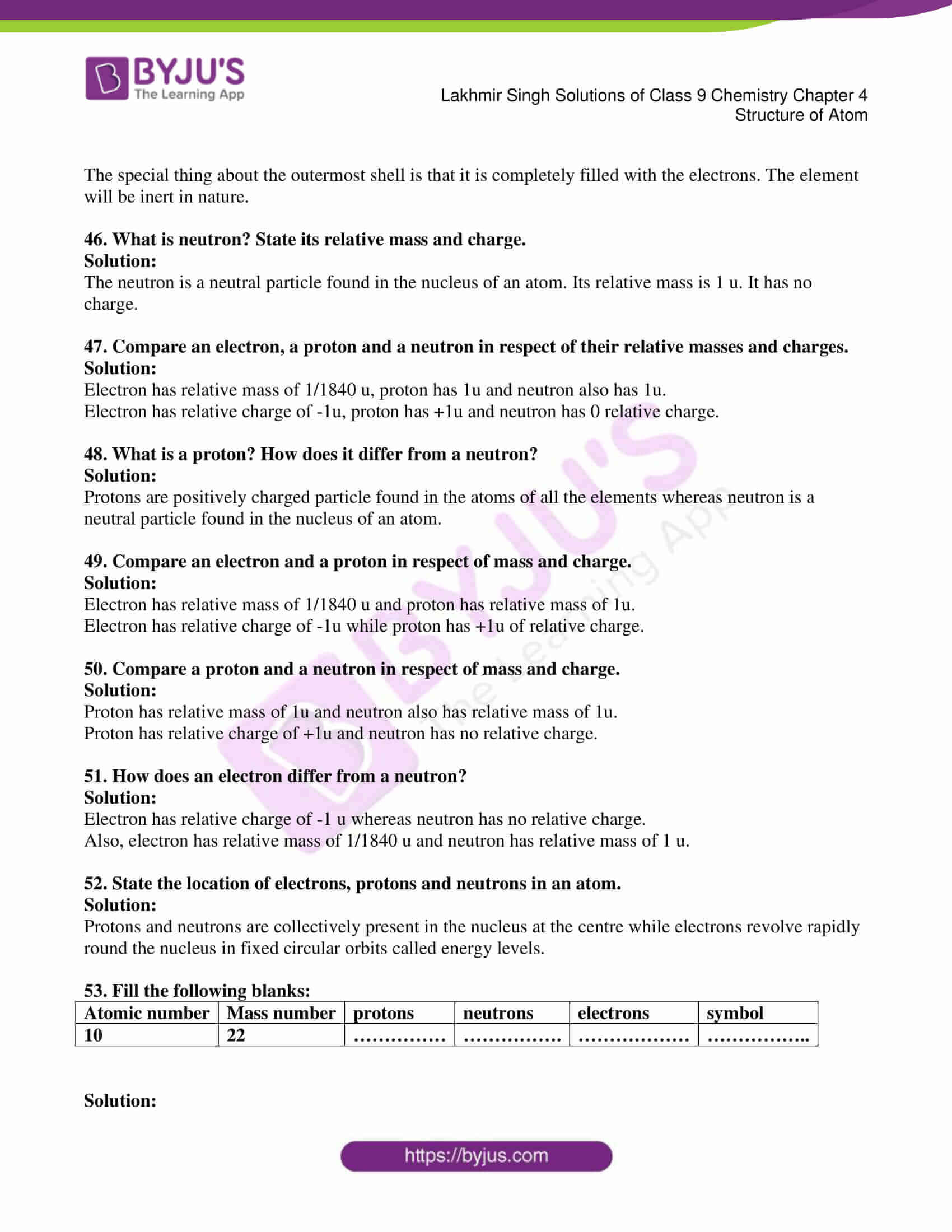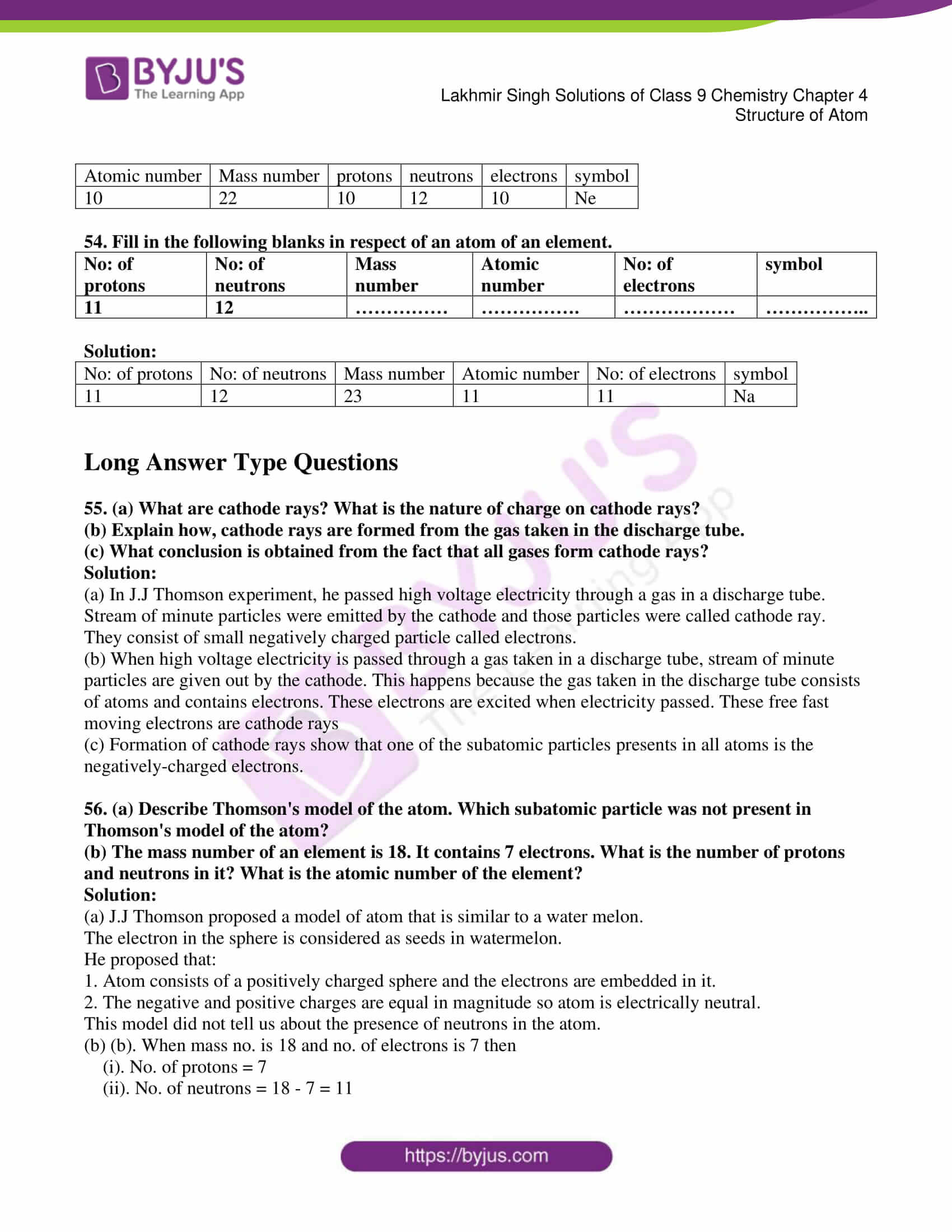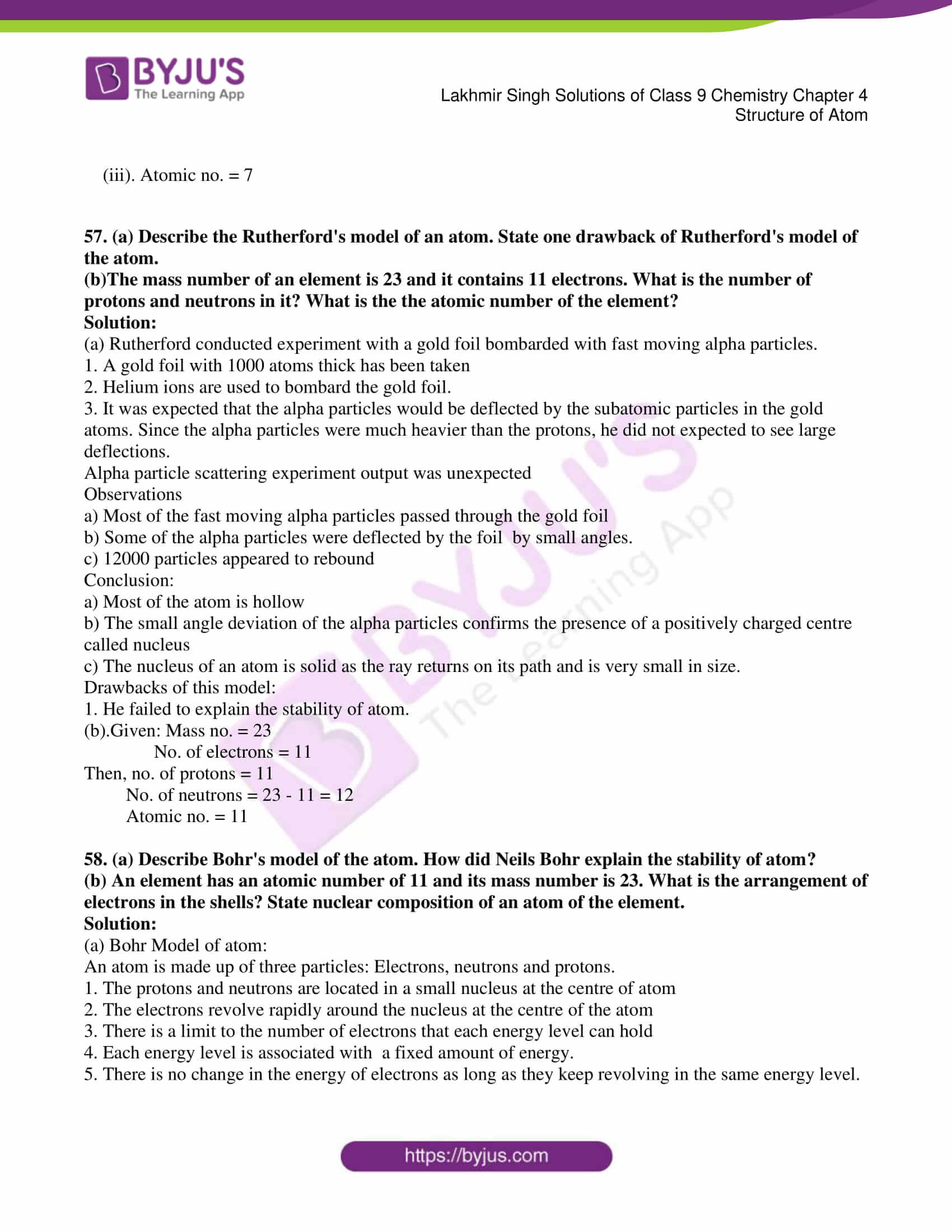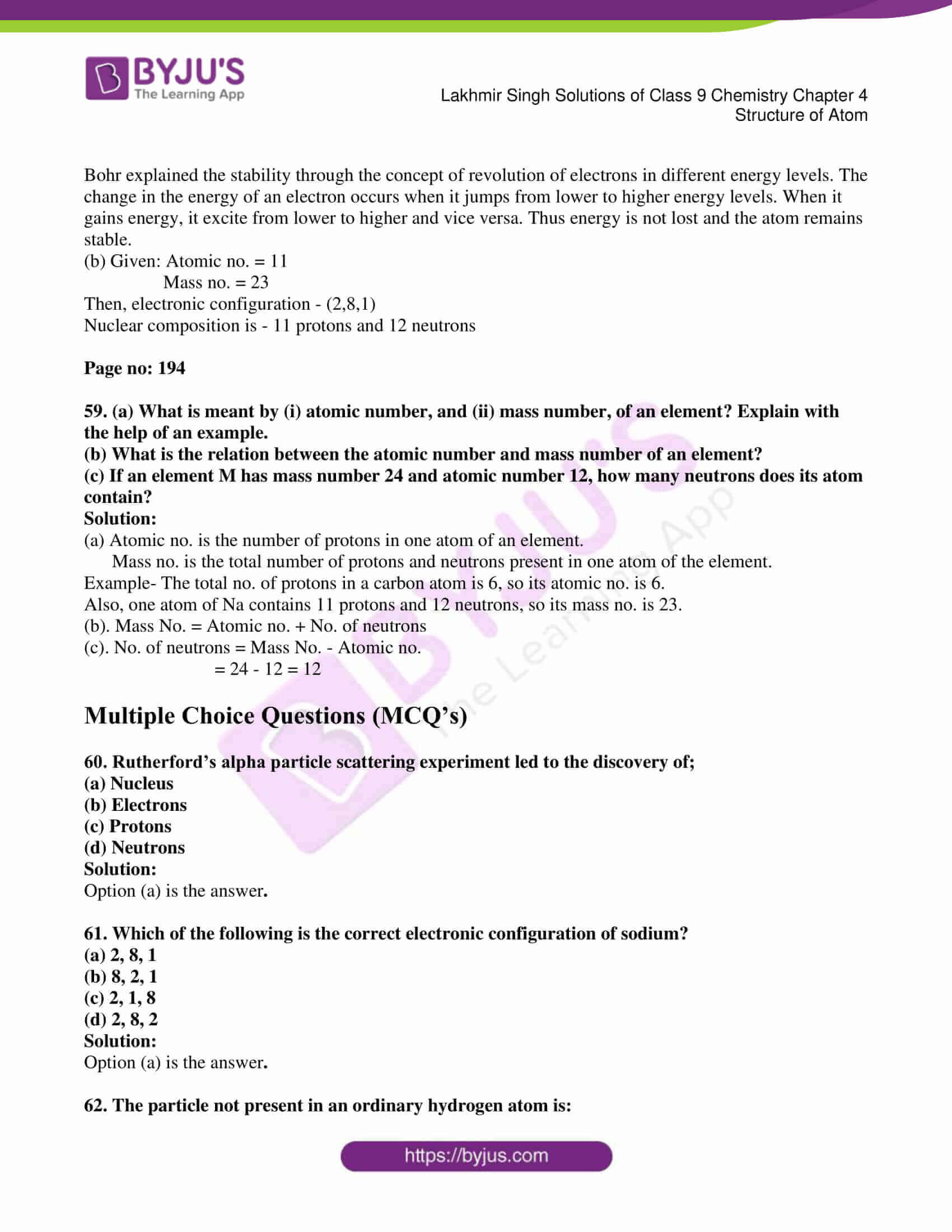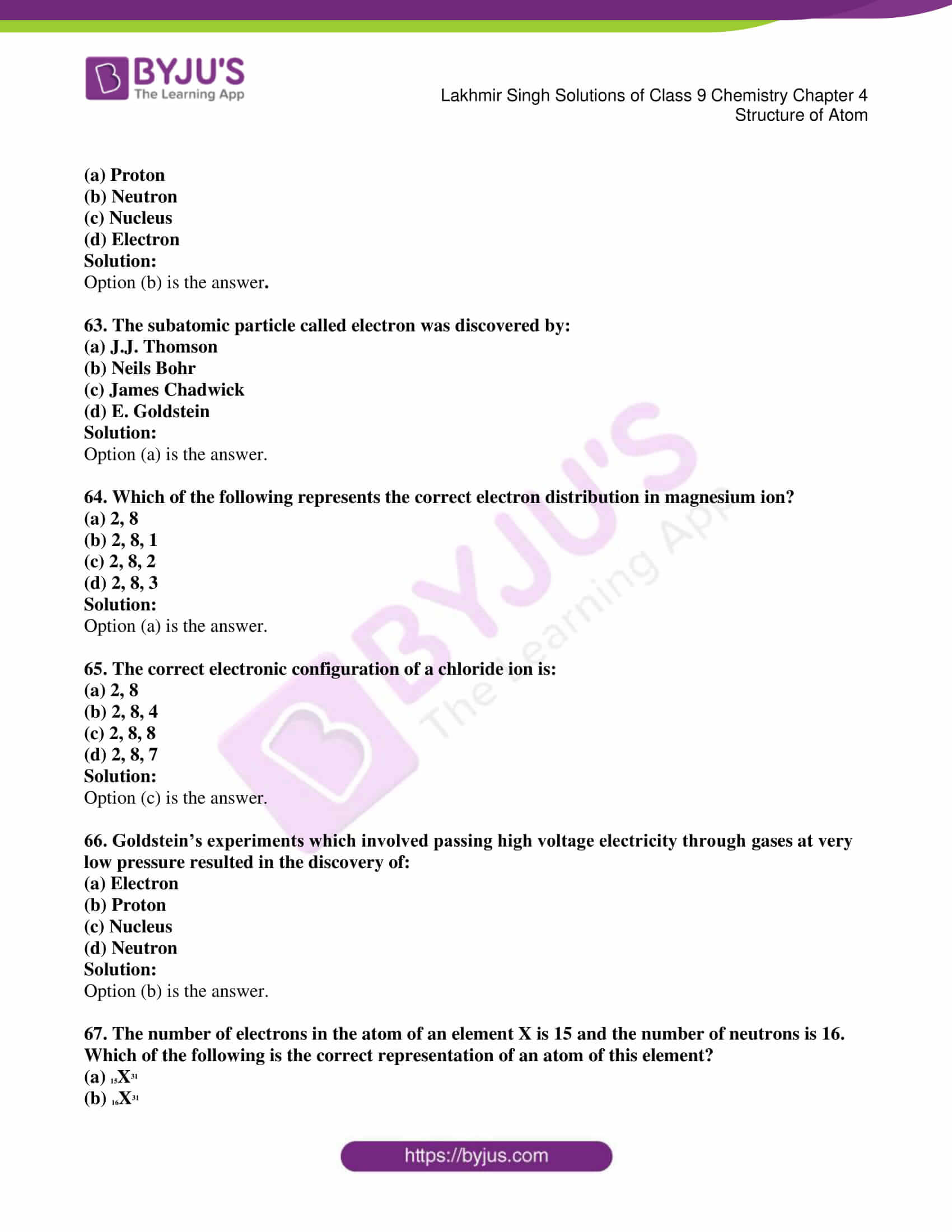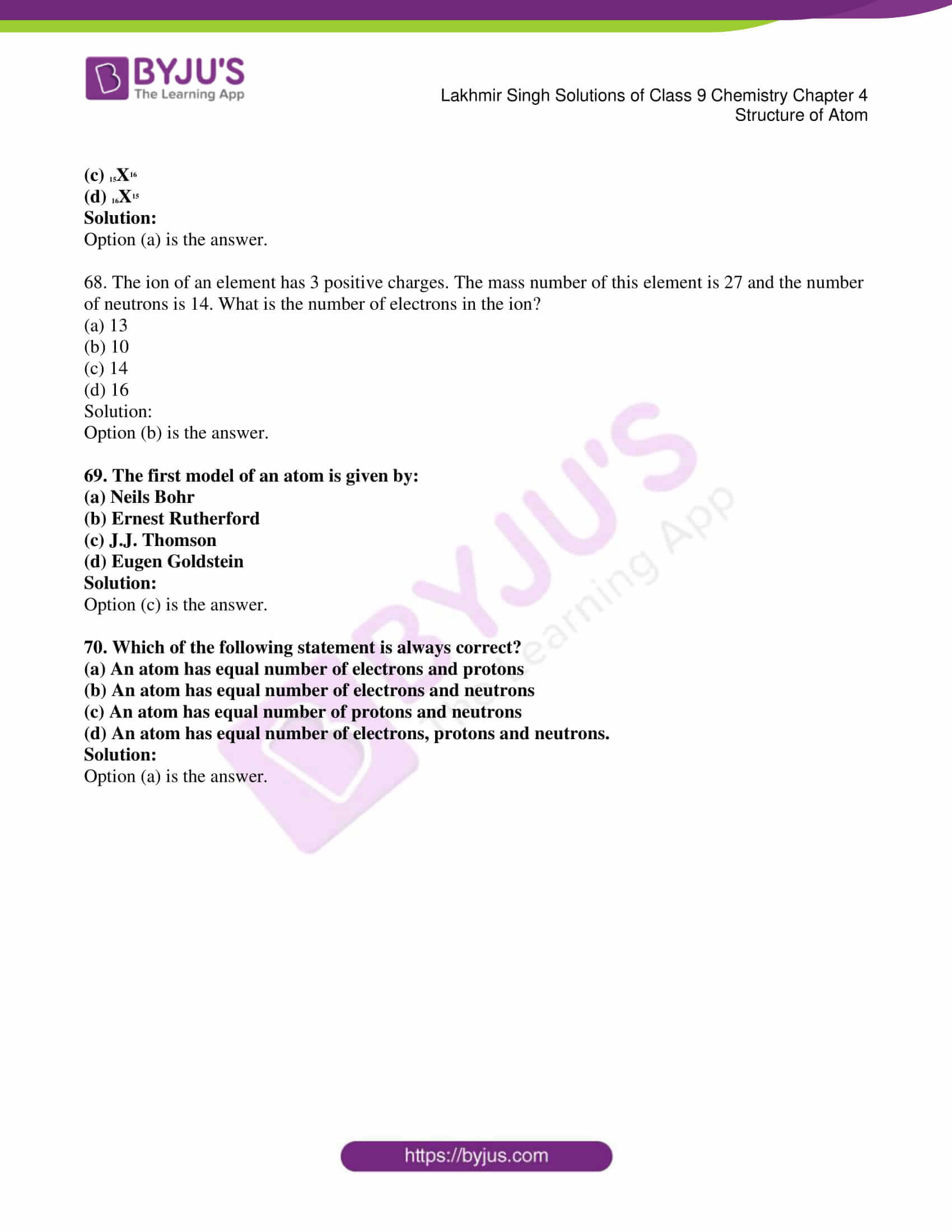### Access Lakhmir Singh Solutions of Class 9 Chemistry Chapter 4 Structure of Atom

Page no: 191

1. Which subatomic particle is not present in an ordinary hydrogen atom?

Solution:

Neutron is not present in ordinary hydrogen atom.

2. Name the scientists who described the arrangement of electrons in an atom.

Solution:

J. J. Thomson described the arrangement of electrons in an atom.

3. What is the maximum number of electrons which can be accommodated in the K shell of an atom?

Solution:

Maximum of 2 e- can be accommodated in K shell of an atom.

4. What is the maximum number of electrons which can be accommodated in the L shell of an atom?

Solution:

Maximum of 8 e- can be accommodated in L shell of an atom.

5. What is the maximum number of electrons an M shell of the atom can accommodate?

Solution:

Maximum of 18 e- can be accommodated in M shell of an atom.

6. What is the maximum number of electrons that can go into the N shell of an atom?

Solution:

Maximum of 32 e- can be accommodated in N shell of an atom.

7. What is the maximum number of electrons which can be accommodated in the:

(a) innermost shell of an atom?

(b) outermost shell of an atom?

Solution:

(a). Maximum of 2 e- can be accommodated in innermost shell of an atom.

(b). Maximum of 8 e- can be accommodated in outermost shell of an atom.

8. Name the three subatomic particles present in an atom.

Solution:

Three subatomic particles present in atom are electrons, protons and neutrons.

9. Name the negatively charged particle present in the atoms of all the elements.

Solution:

Electron is negatively charged particle present in atoms of all the elements.

10. Name the scientist who discovered electron.

Solution:

J. J. Thomson discovered electron.

11. What is the usual symbol for (a) an electron (b) a proton, and (c) a neutron?

Solution 3

(a). e-

(b). p+

(c). n

Page no: 192

12. State whether the following statements are true or false :

(a) Thomson proposed that the nucleus of an atom contains protons and neutrons.

(b) The cathode rays obtained from all the gases consist of negatively charged particles called  electrons.

(c) The anode rays obtained from all the gases consist of positively charged particles called protons.

Solution:

(a) False.

(b) True. The cathode rays obtained from all the gases consist of negatively charged particles called  electrons.

(c) False.

13. Name the central part of an atom where protons and neutrons are held together.

Solution:

Nucleus is the central part of an atom in which protons and neutrons are held together.

14. What are the various letters used by Bohr to represent electron shell in an atom?

Solution:

K, L, M, N were the letters used by Bohr to represent electron shells in an atom.

15. Name the particles which actually determine the mass of an atom.

Solution:

Protons and neutrons actually determine the mass of atom.

16. Name the positively charged particle present in the atoms of all the elements

Solution:

Proton is the positively charged particle present in atoms of all the elements.

17. What is the electronic configuration of a hydrogen atom?

Solution:

Electronic configuration of hydrogen: one as it has one electron in the shell and the atomic number is one.

18. How many times is a proton heavier then an electron?

Solution:

Proton is 1840 times heavier than electron.

19. Name the gas which produces anode rays consisting of protons in the discharge tube experiment.

Solution:

Hydrogen gas produces anode rays consisting of protons in the discharge tube experiment.

20. Which part of an atom was discovered by Rutherford’s alpha particle scattering experiment?

Solution:

Nucleus was discovered by Rutherford in the alpha particle scattering experiment.

21. What is the positive charge on the nucleus of an atom due to?

Solution:

Positive charge on the nucleus is due to presence of protons.

22. State the number of electrons present in the outermost shell of the atoms of the following elements:

(a) Neon                      (b) Chlorine

Solution:

(a) 8 electrons are present in outermost shell of Neon.

(b) 7 electrons are present in outermost shell of Chlorine.

23. Which shell of an atom can accommodate a maximum of?

(a) 8 electrons?                        (b) 32 electrons?

Solution:

(a) L shell can accommodate maximum of 8 e-.

(b) N shell can accommodate maximum of 32 e-.

24. Name the shell of an atom which can accommodate a maximum of :

(a) 2 electrons                 (b) 18 electrons

Solution:

(a) K shell can accommodate maximum of 2 e-.

(b) M shell can accommodate maximum of 18 e-.

25. Which subatomic particle was discovered by :

(i) Chadwick?          (ii)  Thomson?     (iii)  Goldstein?

Solution:

(ii). Thomson discovered ‘electron’.

(iii). Goldstein discovered ‘proton’.

26. Name the subatomic particle whose relative charge is :

(a) +1       (b) -1           (c) 0

Solution;

(a) Proton has relative charge of +1.

(b) Electron has relative charge of -1.

(c) Neutron has relative charge of 0.

27. Fill in the blanks in the following statements:

(a) The number of protons in the nucleus of an atom is called its ______.

(b) The total number of protons and neutrons in the nucleus of an atom is called ______.

(c) An atom has atomic mass number 23 and atomic number 11. The atom has ______ electrons.

(d) An atom of an element has 11 protons, 11 electrons and 12 neutrons. The atomic mass of the atom is ______.

(e) If the nucleus of an atom has atomic number 17 , mass number 37 and there are 17 electrons outside the nucleus, the number of neutrons in it is ______.

(f) Almost all the mass of an atom is concentrated in a small region of space called the ______.

(g) Cathode rays are a beam of fast moving ______.

(h) The anode rays obtained from hydrogen gas consist of particles called ______.

(i) The maximum number of electrons that can be accommodated in L shell are ______.

(j) The maximum number of electrons that can go into the M shell is ______.

(k) The subatomic particle not present in a hydrogen atom is ______.

(l) The electron has ______ charge, the proton has ______ charge, and the neutron has ______ charge.

Solution:

(a)    Atomic number

(b)    Mass number

(c)    11

(d)    23

(e)    20

(f)    Nucleus

(g)    Electrons

(h)    Protons

(i)    8

(j)    18

(k)    Neutron

(l)    Negative; Positive; No

28. What is an electron? State its relative mass and charge.

Solution:

Electron is a negatively charged particle found in the atoms of all elements.

The relative mass of an electron is 1/1840 u.

A charge of -1 is carried by an electron.

29. What is the absolute mass and charge of an electron?

Solution:

Absolute mass of electron is 9 x 10-28 Kg.

Absolute charge on electron is 1.6 x 10-19 C.

30. Give the evidence for the existence of nucleus in an atom.

Solution:

Rutherford experiments give the evidence for the existence of atoms in nucleus

1. Few days got deviated from path.

2. One out of 12000 rays return on their path.

From these observations, he came to a conclusion that

1. Deviation of path shows some positive charge particles

2. The returning back of the ray on its own path shows, there is something which is solid in nature and its number shows the particle is small sized.

31. What important information is furnished about the nucleus of an atom by the alpha particle scattering experiment of Rutherford?

Solution:

Rutherford’s alpha- particle scattering experiment postulates are

(i). Nucleus of an atom is positively charged.

(ii). Nucleus of an atom is very hard and dense.

(iii). Nucleus of an atom is very small as compared to the size of an atom as a whole.

32. How was it shown that an atom has a lot of empty space within it?

Solution:

Rutherford found that most of the alpha particles passed through the gold foil without any deflection and that shows most of the space in the atom is empty.

33. Why is an atom neutral in spite of the presence of charged particles in it?

Solution;

There are equal no. of positive and negative particles in an atom, so it is neutral as a whole.

34. (a) Which of the nuclear particles is present in the same fixed number in the atoms of any particular element?

(b) What do we call this number which is characteristic of a particular element?

Solution:

(a) Proton is present in same fixed no. in the atoms of any particular element.

(b) Atomic no. is characteristic for any particular element.

Page no: 193

35. What is a proton? State its relative mass and charge.

Solution:

Protons are positively charged particle found in the atoms of all the elements.

Relative mass of proton is 1u.

Relative charge of proton is +1 C.

36. What is the absolute mass and charge of a proton?

Solution:

Absolute mass of proton – 1.6x 10-27 Kg

Absolute charge of proton – 1.6 x 10-19 C

37. How does a proton differ from an electron?

Solution:

Proton and neutron differs in –

(1). Proton is positively charged while electron is negatively charged.

(2). Proton is much heavier than electron.

38. State two observations which show that atom is not indivisible.

Solution:

Two observations which shows that atom is not indivisible are-

(1). In J. J. Thomson’s experiment, the stream of cathode rays in the gas discharge tube shows the presence of negatively charged subatomic particles called electrons.

(2). In Goldstein’s experiment, the faint red glow in the gas discharge tube shows the presence of positively charged subatomic particles called protons.

39. All the gases from cathode rays and anode rays when electricity is passed through them:

(i) What does the formation of cathode rays tell us about the atoms?

(ii) What does the formations of anode rays tell us about the atoms?

Solution:

(i) A cathode ray is a stream of high velocity electrons that are produced in a vacuum tube between negatively charged cathode and a positively charged anode. Formation of cathode rays tells about the presence of electrons in all the atoms.

(ii) When a perforated cathode is used in a discharge tube, another set of rays travelling in the direction opposite to that of the cathode rays is seen. These rays are called anode rays. This tells about the protons in all the atoms.

40. What do you understand by the term “electronic configuration” of an element?  Write down the electronic configuration of oxygen (At. No. =8).

Solution:

Electronic configuration of an element is the arrangement of electrons in various shells of an atom of the element.

Electronic configuration of oxygen (atomic no. = 8) is (2,6).

41. An elements has an atomic number 12.How many electrons will be present in the  K,L and M energy shells of its atom?

Solution:

Electronic configuration of element with atomic no. 12- (2,8,2)

K is 2

L is 8

M is 2

42. (a) What is the nucleus of an atom and  what is the nature of charge on it?

(b) Name the scientist who discovered the nucleus of atom.

Solution:

(a). Nucleus is a small positively charged part at the centre of an atom. Nucleus is positively charged.

(b). Rutherford discovered nucleus of an atom.

43. Name the particles used by Rutherford in his experiment on the discovery of nucleus. Also state the charge on these particles.

Solution:

Alpha – particles were used by Rutherford in his experiment on the discovery of nucleus.

Alpha – particles have +2 units of charge.

44. An element has atomic number 13 and an atomic mass of 27.

(a) How many electrons are there in each atom of the element?

(b) How are these electrons distributed in the various energy levels?

Solution:

(a) There will be 13 electrons in each atom of the element. Because in a neutral atom, number of protons is equal to the number of electrons.

(b) Electronic configuration of given element (2, 8, 3) is

K= 2

L=8

M=3

45. Write the distribution of electrons in an atom of element whose atomic number is 18. What is special about the outermost electron shell (or valence shell) of the atom of this element?

Solution:

Atomic No. – 18

Electronic configuration – (2,8,8)

The special thing about the outermost shell is that it is completely filled with the electrons. The element will be inert in nature.

46. What is neutron? State its relative mass and charge.

Solution:

The neutron is a neutral particle found in the nucleus of an atom. Its relative mass is 1 u. It has no charge.

47. Compare an electron, a proton and a neutron in respect of their relative masses and charges.

Solution:

Electron has relative mass of 1/1840 u, proton has 1u and neutron also has 1u.

Electron has relative charge of -1u, proton has +1u and neutron has 0 relative charge.

48. What is a proton? How does it differ from a neutron?

Solution:

Protons are positively charged particle found in the atoms of all the elements whereas neutron is a neutral particle found in the nucleus of an atom.

49. Compare an electron and a proton in respect of mass and charge.

Solution:

Electron has relative mass of 1/1840 u and proton has relative mass of 1u.

Electron has relative charge of -1u while proton has +1u of relative charge.

50. Compare a proton and a neutron in respect of mass and charge.

Solution:

Proton has relative mass of 1u and neutron also has relative mass of 1u.

Proton has relative charge of +1u and neutron has no relative charge.

51. How does an electron differ from a neutron?

Solution:

Electron has relative charge of -1 u whereas neutron has no relative charge.

Also, electron has relative mass of 1/1840 u and neutron has relative mass of 1 u.

52. State the location of electrons, protons and neutrons in an atom.

Solution:

Protons and neutrons are collectively present in the nucleus at the centre while electrons revolve rapidly round the nucleus in fixed circular orbits called energy levels.

53. Fill the following blanks:

 Atomic number Mass number protons neutrons electrons symbol 10 22 …………… ……………. ……………… ……………..

Solution:

 Atomic number Mass number protons neutrons electrons symbol 10 22 10 12 10 Ne

54. Fill in the following blanks in respect of an atom of an element.

 No: of protons No: of neutrons Mass number Atomic number No: of electrons symbol 11 12 …………… ……………. ……………… ……………..

Solution:

 No: of protons No: of neutrons Mass number Atomic number No: of electrons symbol 11 12 23 11 11 Na

55. (a) What are cathode rays? What is the nature of charge on cathode rays?

(b) Explain how, cathode rays are formed from the gas taken in the discharge tube.

(c) What conclusion is obtained from the fact that all gases form cathode rays?

Solution:

(a) In J.J Thomson experiment, he passed high voltage electricity through a gas in a discharge tube. Stream of minute particles were emitted by the cathode and those particles were called cathode ray. They consist of small negatively charged particle called electrons.

(b) When high voltage electricity is passed through a gas taken in a discharge tube, stream of minute particles are given out by the cathode. This happens because the gas taken in the discharge tube consists of atoms and contains electrons. These electrons are excited when electricity passed. These free fast moving electrons are cathode rays

(c) Formation of cathode rays show that one of the subatomic particles presents in all atoms is the negatively-charged electrons.

56. (a) Describe Thomson’s model of the atom. Which subatomic particle was not present in Thomson’s model of the atom?

(b) The mass number of an element is 18. It contains 7 electrons. What is the number of protons and neutrons in it? What is the atomic number of the element?

Solution:

(a) J.J Thomson proposed a model of atom that is similar to a water melon.

The electron in the sphere is considered as seeds in watermelon.

He proposed that:

1. Atom consists of a positively charged sphere and the electrons are embedded in it.

2. The negative and positive charges are equal in magnitude so atom is electrically neutral.

This model did not tell us about the presence of neutrons in the atom.

(b) (b). When mass no. is 18 and no. of electrons is 7 then

(i). No. of protons = 7

(ii). No. of neutrons = 18 – 7 = 11

(iii). Atomic no. = 7

57. (a) Describe the Rutherford’s model of an atom. State one drawback of Rutherford’s model of the atom.

(b)The mass number of an element is 23 and it contains 11 electrons. What is the number of protons and neutrons in it? What is the the atomic number of the element?

Solution:

(a) Rutherford conducted experiment with a gold foil bombarded with fast moving alpha particles.

1. A gold foil with 1000 atoms thick has been taken

2. Helium ions are used to bombard the gold foil.

3. It was expected that the alpha particles would be deflected by the subatomic particles in the gold atoms. Since the alpha particles were much heavier than the protons, he did not expected to see large deflections.

Alpha particle scattering experiment output was unexpected

Observations

a) Most of the fast moving alpha particles passed through the gold foil

b) Some of the alpha particles were deflected by the foil  by small angles.

c) 12000 particles appeared to rebound

Conclusion:

a) Most of the atom is hollow

b) The small angle deviation of the alpha particles confirms the presence of a positively charged centre called nucleus

c) The nucleus of an atom is solid as the ray returns on its path and is very small in size.

Drawbacks of this model:

1. He failed to explain the stability of atom.

(b).Given: Mass no. = 23

No. of electrons = 11

Then, no. of protons = 11

No. of neutrons = 23 – 11 = 12

Atomic no. = 11

58. (a) Describe Bohr’s model of the atom. How did Neils Bohr explain the stability of atom?

(b) An element has an atomic number of 11 and its mass number is 23. What is the arrangement of electrons in the shells? State nuclear composition of an atom of the element.

Solution:

(a) Bohr Model of atom:

An atom is made up of three particles: Electrons, neutrons and protons.

1. The protons and neutrons are located in a small nucleus at the centre of atom

2. The electrons revolve rapidly around the nucleus at the centre of the atom

3. There is a limit to the number of electrons that each energy level can hold

4. Each energy level is associated with  a fixed amount of energy.

5. There is no change in the energy of electrons as long as they keep revolving in the same energy level.

Bohr explained the stability through the concept of revolution of electrons in different energy levels. The change in the energy of an electron occurs when it jumps from lower to higher energy levels. When it gains energy, it excite from lower to higher and vice versa. Thus energy is not lost and the atom remains stable.

(b) Given: Atomic no. = 11

Mass no. = 23

Then, electronic configuration – (2,8,1)

Nuclear composition is – 11 protons and 12 neutrons

Page no: 194

59. (a) What is meant by (i) atomic number, and (ii) mass number, of an element? Explain with the help of an example.

(b) What is the relation between the atomic number and mass number of an element?

(c) If an element M has mass number 24 and atomic number 12, how many neutrons does its atom contain?

Solution:

(a) Atomic no. is the number of protons in one atom of an element.

Mass no. is the total number of protons and neutrons present in one atom of the element.

Example- The total no. of protons in a carbon atom is 6, so its atomic no. is 6.

Also, one atom of Na contains 11 protons and 12 neutrons, so its mass no. is 23.

(b). Mass No. = Atomic no. + No. of neutrons

(c). No. of neutrons = Mass No. – Atomic no.

= 24 – 12 = 12

Multiple Choice Questions (MCQ’s)

60. Rutherford’s alpha particle scattering experiment led to the discovery of;

(a) Nucleus

(b) Electrons

(c) Protons

(d) Neutrons

Solution:

61. Which of the following is the correct electronic configuration of sodium?

(a) 2, 8, 1

(b) 8, 2, 1

(c) 2, 1, 8

(d) 2, 8, 2

Solution:

62. The particle not present in an ordinary hydrogen atom is:

(a) Proton

(b) Neutron

(c) Nucleus

(d) Electron

Solution:

63. The subatomic particle called electron was discovered by:

(a) J.J. Thomson

(b) Neils Bohr

(d) E. Goldstein

Solution:

64. Which of the following represents the correct electron distribution in magnesium ion?

(a) 2, 8

(b) 2, 8, 1

(c) 2, 8, 2

(d) 2, 8, 3

Solution:

65. The correct electronic configuration of a chloride ion is:

(a) 2, 8

(b) 2, 8, 4

(c) 2, 8, 8

(d) 2, 8, 7

Solution:

66. Goldstein’s experiments which involved passing high voltage electricity through gases at very low pressure resulted in the discovery of:

(a) Electron

(b) Proton

(c) Nucleus

(d) Neutron

Solution:

67. The number of electrons in the atom of an element X is 15 and the number of neutrons is 16. Which of the following is the correct representation of an atom of this element?

(a) 15X31

(b) 16X31

(c) 15X16

(d) 16X15

Solution:

68. The ion of an element has 3 positive charges. The mass number of this element is 27 and the number of neutrons is 14. What is the number of electrons in the ion?

(a) 13

(b) 10

(c) 14

(d) 16

Solution:

69. The first model of an atom is given by:

(a) Neils Bohr

(b) Ernest Rutherford

(c) J.J. Thomson

(d) Eugen Goldstein

Solution:

70. Which of the following statement is always correct?

(a) An atom has equal number of electrons and protons

(b) An atom has equal number of electrons and neutrons

(c) An atom has equal number of protons and neutrons

(d) An atom has equal number of electrons, protons and neutrons.

Solution:

Also, visit Lakhmir Singh Solutions for Class 9 Chemistry to get complete solutions for all chapters.